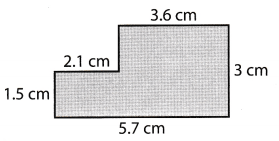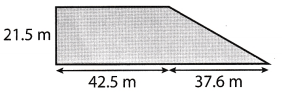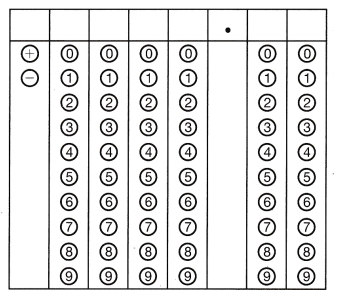# Texas Go Math Grade 6 Benchmark Test Answer Key Part 2

Refer to our Texas Go Math Grade 6 Answer Key Pdf to score good marks in the exams. Test yourself by practicing the problems from Texas Go Math Grade 6 Benchmark Test Answer Key Part 2.

## Texas Go Math Grade 6 Benchmark Test Answer Key Part 2

Selected Response

Question 1.
What is the prime factorization of 300?
(A) 2 × 3 × 5
(B) 3 × 4 × 52
(C) 3 × 10
(D) 22 × 3 × 52
(D) 22 × 3 × 52

Explanation:
We will, write prime factorization of 300 in order to pick right answer;
300 = 2 ∙ 150
= 2 ∙ 2 ∙ 75
= 22 ∙ 3 ∙ 25
= 22 ∙ 3 ∙ 5 ∙ 5
= 22 ∙ 3 ∙ 52

Question 2.
Wilson bought gift cards for some lawyers and their assistants. Each lawyer got a gift card worth $l. Each assistant got a gift card worth$o. There are 12 lawyers. Each lawyer has two assistants. The expression for the total cost of the gift cards is 12l + 24a. Write an expression that is equivalent to the given expression.
(A) 12(l + 2 a)
(B) 12(l + 3a)
(C) 12(l + 24a)
(D) 24(l + 2a)
(A) 12(l + 2 a)

Explanation:
We can notice that answer A is correct:
12(l + 2a) = 12(l) + 12(2a)
= 12l + 24a

Question 3.
A triangle has sides with lengths of 2x – 7, 5x – 3, and 2x – 2. What is the perimeter of the triangle?
(A) 9x – 12
(B) 5x -12
(C) -x – 6
(D) -3x
(A) 9x – 12

Explanation:
The perimeter of the triangle is sum of Lengths of all three sides. In this case it is:
2x – 7+ (5x – 3) + (2x – 2) = 2x – 7 + 5x – 3 + 2x – 2
= 9x – 12
Conclusion is the perimeter of given triangle is 9x – 12.

Question 4.
Brian is ordering tickets online for a concert. The price of each ticket for the concert is $f. For online orders, there is an additional charge of$11 per ticket and a service charge of $14 for the entire order. The cost for 7 tickets purchased online can be represented by the expression 7(t + 11) + 14. Which expression is equivalent to the cost expression? (A) 18t + 14 (B) 7t + 91 (C) 32t (D) 7t + 25 Answer: (B) 7t + 91 Explanation: We wiLl simplify given expression: 7(t) + 7(11) + 14 = 7t + 77 + 14 = 7t + 91 Conclusion is that 7t + 91 is expression which is equivalent to 7(t + 11) + 14. Question 5. A driveway is 81 feet long, 12 feet wide, and 6 inches deep. How many cubic feet of concrete will be required for the driveway? (A) 423 cubic feet (B) 458 cubic feet (C) 486 cubic feet (D) 5,832 cubic feet Answer: (D) 5,832 cubic feet Explanation: We will calculate how many cubic feet of concrete will, be required for the driveway by multiplying length, width and deepth of a driveway and get: 81 ∙ 12 ∙ 6 = 5832 So, it will be required 5832 cubic feet of concrete for the driveway. Question 6. What is the area of the polygon?(A) 3.15 cm2 (B) 10.8 cm2 (C) 13.95 cm2 (D) 1,395 cm2 Answer: (C) 13.95 cm2 Explanation: We will, separate this polygon on two rectangular. First has one side 1.5 cm long and the other side is 2.1 cm. The second has one side of 3.6 cm and the other is 3 cm. We will calculate areas of both rectangular and their sum will be the area of polygon. So, we have the following: 1st rectangular: area = 1.5 ∙ 2.1 = 3.15 cm2 2nd rectangular: area= 3.6 ∙ 3 = 10.8cm2 So, area of polygon is 3.15 + 10.8 = 13.95 cm2. Conclusion is that correct answer is C. Question 7. What is the area of the polygon?(A) 861.075 m2 (B) 1,317.95 m2 (C) 1,722.15 m2 (D) 2,635.9 m2 Answer: (B) 1,317.95 m2 Explanation: This polygon we can split on one rectangular whose sides are 21.5 m and 42.5 m and on one right triangle whose legs are 21.5 m and 37.6 m We will calculate their areas and their sum will be area of the given polygon. First we wilt calculate area of rectangular: area= 21.5 ∙ 42.5 = 913.75m2 And now we will calculate area of right triangle: area = $$\frac{21.5 \cdot 37.6}{2}$$ = 404.2m2 And now, we wilt find area of polygon as the sum of those two areas: area of the polygon 913.75 + 404.2 = 1317.95m2 So, correct answer is B Question 8. Kahlil is recording a rhythm track for a new song that he is working on. He wants the track to be more than 10 seconds long. His friend tells him the track needs to be at least 5 seconds longer than that to match the lyrics he has written. Write an inequality to represent the track’s length in seconds. (A) t > 5 (B) t > 15 (C) t < 5 (D) t < 10 Answer: (B) t > 15 Explanation: Because the track needs to be at least 5 seconds longer than 10 seconds to match the lyrics he has written, conclusion is the following: t > 10 + 5 = 15 So, it must be longer than 15 seconds at least, correct answer is B. Question 9. In a fish tank, $$\frac{8}{11}$$ of the fish have a red stripe on them. If 16 of the fish have red stripes, how many total fish are in the tank? (A) 21 fish (B) 22 fish (C) 20 fish (D) 26 fish Answer: (B) 22 fish Explanation: We know that $$\frac{8}{11}$$ of the total fish which are in the tank, x, have a red stripe on them and that number is 16. So, we have the following equation, and solving it for x, we will find number of total fish in the tank. $$\frac{8}{11}$$x = 16 8x = 176 x = $$\frac{176}{8}$$ x = 22 So, there are 22 fish in the tank. Correct answer is B. Question 10. Solve the equation u × 5.6 = 6.16. (A) u = 34.5 (B) u = 1 (C) u = 1.1 (D) u = 0.77 Answer: (C) u = 1.1 Explanation: In order to solve this equation for u, we will divide result by 5.6 and get: u = $$\frac{6.16}{5.6}$$ = 1.1 So, the right answer is C. Question 11. It will be Lindsay’s birthday soon, and her friends Chris, Mikhail, Wolfgang, and Adrian have contributed equal amounts of money to buy her a present. They have a total of$27.00 to spend. Write an equation that models the situation and find the amount each friend contributed.
(A) 4x = $27.00; x =$7.75
(B) 5x = $27.00; x =$5.40
(C) 4x = $27.00; x =$108.00
(D) 4x = $27.00; x =$6.75
(D) 4x = $27.00; x =$6.75

Explanation:
Because there are 4 friends and they gave equal amount of money and have in total $27, the equation which describes this situation is: 4x =$27
We wiLl solve this equation for x dividing 27 by 4 and get:
x = 6.75

Question 12.
Write an equation for the function. Tell what each variable you use represents. A plant’s height is 1.4 times its age.
(A) x = plant’s height; y = plant’s age; x = 1.4y
(B) x = plant’s age; y = plant’s height; x = 1.4y
(C) x = plant’s height; y = plant’s age; y = 1.4x
(D) x = plant’s height; y = plant’s age; 1.4 = xy
(A) x = plant’s height; y = plant’s age; x = 1.4y

Explanation:
If we Let x represents height and y represents plant’s age required equation would be:
x = 1.4y

Question 13.
Jared is redoing his bathroom floor with tiles measuring 6 in. by 14 in. The floor has an area of 8,900 in2. What is the least number of tiles he will need?
(A) 105 tiles
(B) 105.95 tiles
(C) 106 tiles
(D) 445 tiles
(B) 105.95 tiles

Explanation:
First we will calculate an area of one tile.
area of tile= 6 14 = 84 in2
Now, we will divide area of the floor by area of one tile in order to get required number of tiles which are needed for redoing bathroom.
$$\frac{8900}{84}$$ = 105.95
So, Jared will need 105.95 tiles for redoing bathroom.

Question 14.
Mrs. Rissoto filled her daughter’s plastic swimming pool with water. The water level in the pool changed by 8 gallons each hour due to a small hole in the bottom of the pool. After 6 hours, the pool contained 132 gallons of water. How much water was in the pool originally?
(A) 180 gallons
(B) 140 gallons
(C) 124 gallons
(D) 84 gallons
(A) 180 gallons

Explanation:
On 132 gallons of water we have to add quantity of water which went throw a small holl.
That quantity is:
6 ∙ 8 = 48
So, there was 132 + 48 = 180 gallons of water originally in the pool.

Question 15.
What is the range of the data represented by the box-and-whisker plot?(A) 25
(B) 30
(C) 35
(D) 60

Question 16.
In a box-and-whisker plot, the interquartile range is a measure of the spread of the middle half of the data. Find the interquartile range for the data set 10, 3, 7, 5, 9, 12, 15.
(A) 12
(B) 8
(C) 7
(D) 6

Question 17.
What is the area of the base of a rectangular prism with a height of 10 centimeters and a volume of 400 cubic centimeters?
(A) 4 square centimeters
(B) 40 square centimeters
(C) 400 square centimeters
(D) 4,000 square centimeters
(B) 40 square centimeters

Explanation:
Formula for calculating volume of prism is V = BH. where B is area of the base and H is height
In this task we have volume and height. Area of the base we will find dividing volume by height.
So, we have following:
B = $$\frac{400}{10}$$ = 40 cm2
To find the mileage, or how many miles a car can travel per gallon of gasoline, you can use the expression $$\frac{m}{g}$$, where m is the distance in miles and g is the number of gallons of gas used. Find the gas mileage in miles per gallon for a car that travels 212 miles on 8 gallons of gas.Because $$\frac{m}{g}$$ is formula for finding the mileage where m is the distance in miles and g is the number of gallons of gas used, and in this task we are given the distance in miles and number of gallons.
mileage = $$\frac{212}{8}$$ = 26.5